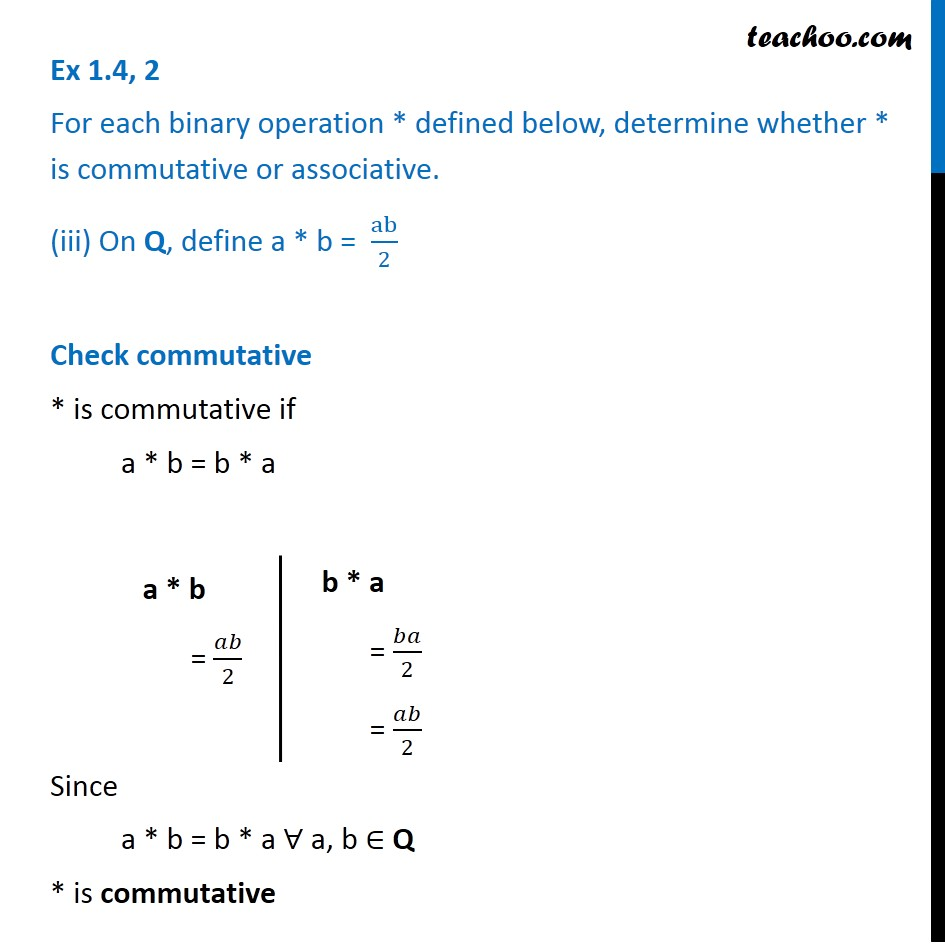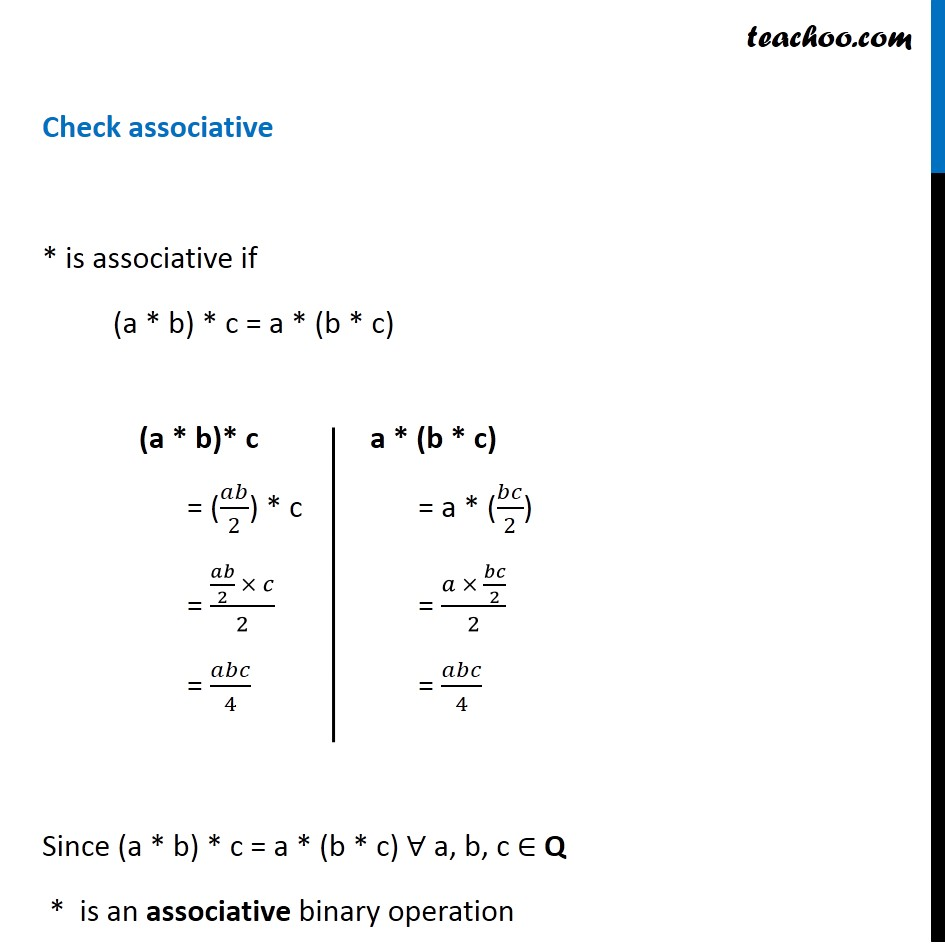Binary Operations

Chapter 1 Class 12 Relation and Functions
Serial order wiseLearn in your speed, with individual attention - Teachoo Maths 1-on-1 Class

### Transcript

Ex 1.4, 2 For each binary operation * defined below, determine whether * is commutative or associative. (iii) On Q, define a * b = ab/2 Check commutative * is commutative if a * b = b * a Since a * b = b * a ∀ a, b ∈ Q * is commutative a * b = 𝑎𝑏/2 b * a = 𝑏𝑎/2 = 𝑎𝑏/2 Check associative * is associative if (a * b) * c = a * (b * c) Since (a * b) * c = a * (b * c) ∀ a, b, c ∈ Q * is an associative binary operation (a * b)* c = (𝑎𝑏/2) * c = (𝑎𝑏/2 × 𝑐)/2 = 𝑎𝑏𝑐/4 a * (b * c) = a * (𝑏𝑐/2) = (𝑎 × 𝑏𝑐/2)/2 = 𝑎𝑏𝑐/4